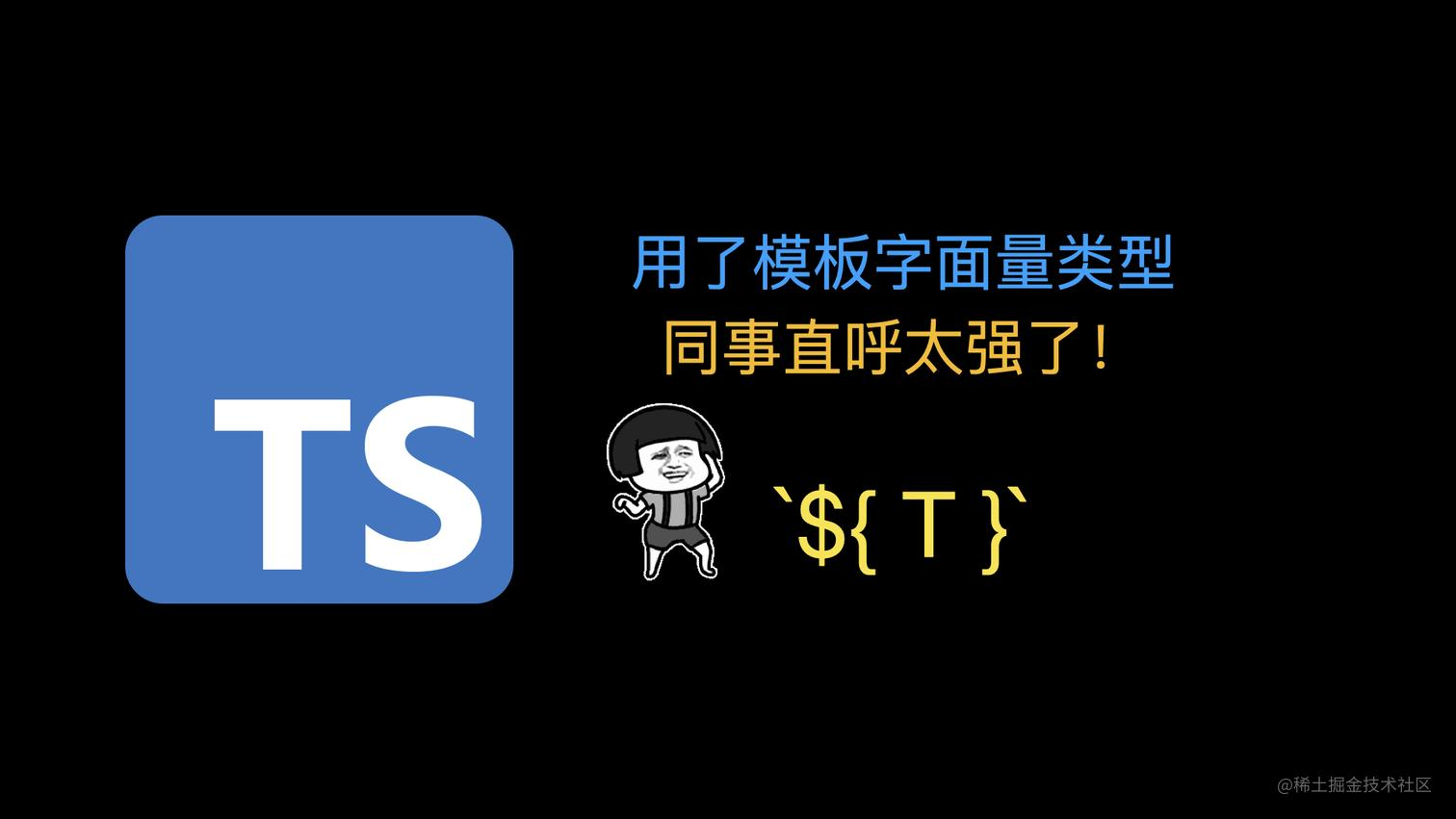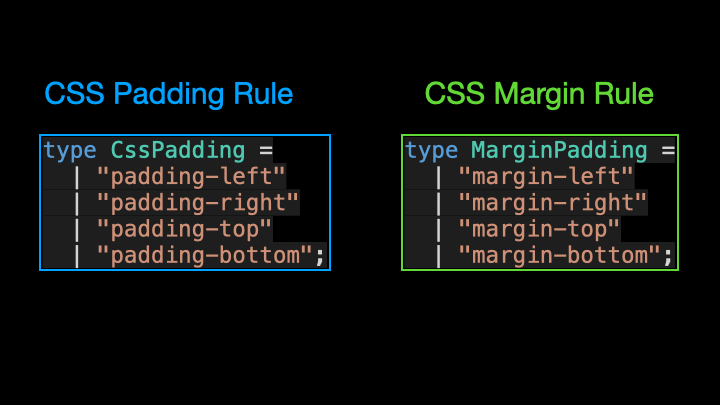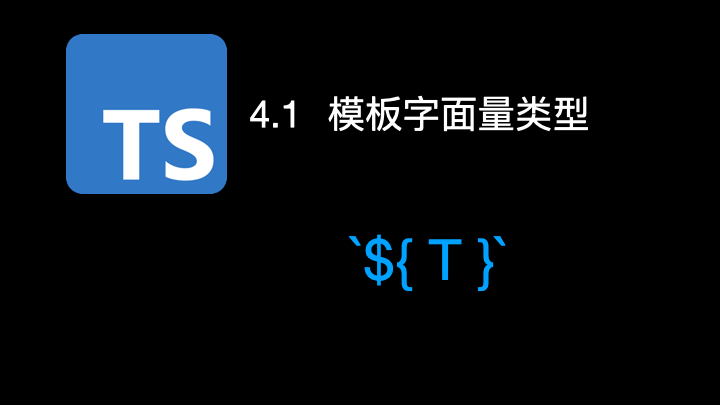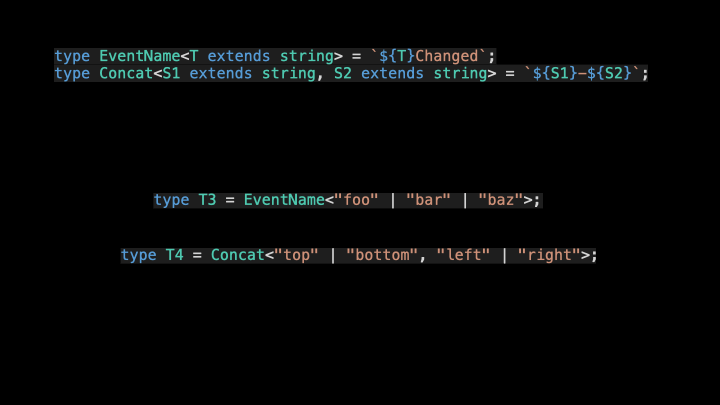# 用了模板字面量类型，同事直呼太强了！12.7W 播放量的 TS 动画版进阶教程合集来了！，通过形象生动的动画，让你轻松搞懂 TypeScript 的难点和核心知识点！type CssPadding =

type MarginPadding =
| "margin-left"
| "margin-right"
| "margin-top"
| "margin-bottom";type Direction = "left" | "right" | "top" | "bottom";
​
type CssPadding = padding-${Direction}; type MarginPadding = margin-${Direction};type Concat<S1 extends string, S2 extends string> = ${S1}-${S2};
type ToString<T extends string | number | boolean | bigint> = ${T}; ​ type T0 = EventName<"foo">; // 'fooChanged' type T1 = Concat<"Hello", "World">; // 'Hello-World' type T2 = ToString<"阿宝哥" | 666 | true | -1234n>; // "阿宝哥" | "true" | "666" | "-1234" 复制代码对于上述的例子来说，其实并不复杂。但现在问题来了，如果传入 EventName 或 Concat 工具类型的实际类型是联合类型的话，那么结果又会是怎样呢？接下来，我们来验证一下： type T3 = EventName<"foo" | "bar" | "baz">; // "fooChanged" | "barChanged" | "bazChanged" type T4 = Concat<"top" | "bottom", "left" | "right">; // "top-left" | "top-right" | "bottom-left" | "bottom-right" 复制代码为什么会生成这样的类型呢？这是因为对于模板字面量类型来说，当类型占位符的实际类型是联合类型（A ｜B ｜C）的话，就会被自动展开： [${A|B|C}] => [${A}] | [${B}] | [${C}] 复制代码而对于包含多个类型占位符的情形，比如 Concat 工具类型。多个占位符中的联合类型解析为叉积： ${A|B}-${C|D} => ${A}-${C} | ${A}-${D} | ${B}-${C} | ${B}-${D} 复制代码了解完上述的运算规则，你应该就能理解生成的 T3 和 T4 类型了。在使用模板字面量类型的过程中，我们还可以使用 TypeScript 提供的，用于处理字符串类型的内置工具类型，比如 UppercaseLowercaseCapitalizeUncapitalize。具体的使用方式是这样的： type GetterName<T extends string> = get${Capitalize<T>};
type Cases<T extends string> = ${Uppercase<T>}${Lowercase<T>} ${Capitalize<T>}${Uncapitalize<T>};
​
type T5 = GetterName<'foo'>;  // "getFoo"
type T6 = Cases<'bar'>;  // "BAR bar Bar bar"

type Direction = "left" | "right" | "top" | "bottom";
type InferRoot<T> = T extends ${infer R}${Capitalize<Direction>} ? R : T;
​
type T7 = InferRoot<"marginRight">; // "margin"

type MappedTypeWithNewKeys<T> = {
[K in keyof T as NewKeyType]: T[K]
//            ^^^^^^^^^^^^^
//            This is the new syntax!
}type Getters<T> = {
[K in keyof T as get${Capitalize<string & K>}]: () => T[K] }; ​ interface Person { name: string; age: number; location: string; } ​ type LazyPerson = Getters<Person>; // { // getName: () => string; // getAge: () => number; // getLocation: () => string; // } 复制代码在以上代码中，因为 keyof T 返回的类型可能会包含 symbol 类型，而 Capitalize 工具类型要求处理的类型需要是 string 类型的子类型，所以需要通过交叉运算符进行类型过滤。除了实现简单的工具类型之外，我们还可以实现比较复杂的工具类型。比如，用于获取对象类型中，任意层级属性的类型。 type PropType<T, Path extends string> = string extends Path ? unknown : Path extends keyof T ? T[Path] : Path extends ${infer K}.\${infer R}
? K extends keyof T
? PropType<T[K], R>
: unknown
: unknown;
​
declare function getPropValue<T, P extends string>(
obj: T,
path: P
): PropType<T, P>;
​
const obj = { a: { b: { c: 666, d: "阿宝哥" } } };
let a = getPropValue(obj, "a"); // { b: {c: number, d: string } }
let ab = getPropValue(obj, "a.b"); // {c: number, d: string }
let abd = getPropValue(obj, "a.b.d"); // string